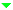Optical Materials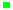Optical GlassLow Expansion MaterialsIR MaterialsCrystals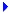Micro-Optical ComponentsFiber Optic ComponentsLight Sources & PhotodiodesWafersServices

Potassium Titanyl  Phosphate (KTiOPO4 or KTP)

KTP is a nonlinear optical (NLO) material which is known for its high NLO coefficient, high electro-optical coefficient, wide transparency range, small walk off angle, good chemical and mechanical properties, and a moderate optical damage threshold. These properties make KTP very attractive for different nonlinear optical applications.

Applications:

• Frequency Doubling (SHG) of Nd-doped Lasers for Green/Red Output
• Frequency Mixing of Nd Laser and Diode Laser for Blue Output
• Parametric Sources (OPG, OPA and OPO) for 0.6mm-4.5mm Tunable Output
• E-O Modulators and Optical Switches

Phase Matching Angles:

Figure 1 & 2 show type II SHG phase matching angles vs. fundamental wavelengths in both XY and XZ planes. In XY plane the slope ∂(Δk)/∂θ is small, which ensures a large acceptance angle and a small walk off. In XZ plane the slope ∂(Δk)/∂λ is almost zero around the wavelength 2.0um, which ensures a large spectral acceptance. These two techniques are known as non-critical phase matching (NCPM).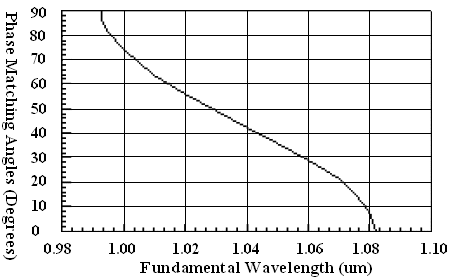Figure 1: KTP type II phase-matching angles vs. wavelengths in XY plane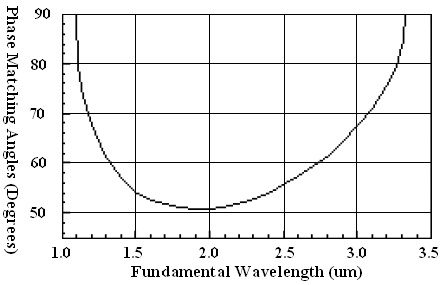Figure 2: KTP type II phase-matching angles vs. wavelengths in XZ plane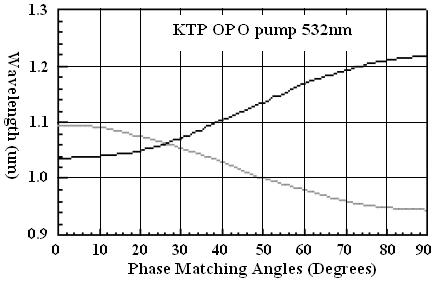Figure 3: KTP OPO tuning curve in XY plane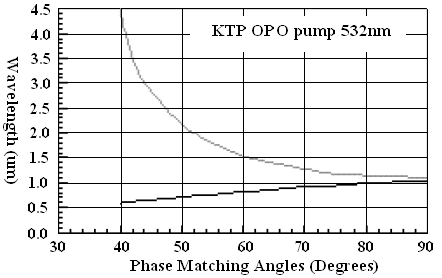Figure 4: KTP OPO tuning curve in XZ plane

Figure 3 & 4 show OPO tuning curve in both XY and XZ planes with a pump wavelength 532nm. As an efficient OPO crystal, KTP generates tunable parametric sources from visible (0.6um) to mid-IR (4.5um). Due to the development of NCPM, KTP OPO/OPA pumped by a tunable laser such as Ti:Sapphire could generates new wavelengths from 1.04um to 1.45um at a fixed KTP orientation.

 Crystal Structure Orthorhombic lattice constant (Å) a = 6.404, b = 10.616, c = 12.814 Melting Point (°C) 1172 Mohs Hardness ≈ 5 Density (g/cm^3) 3.02 Absorption Coefficient (/cm) α<0.001  at 1064nm & 532nm Hygroscopic Susceptibility No Relative Dielectric Constant εeff = 13 Linear Coefficient of Thermal Expansion (100°C)  ( /K) a1: 8.7X10-6a2: 10.5X10-6a3: -0.2X10-6 Transmission Range (nm) 350~4500 Damage Threshold (MW/cm2) 10  at 1064nm Constant of Dispersion (dn/dT)  (/°C) dnx/dT = 1.1X10-5dny/dT = 1.3X10-5 dnz/dT = 1.6X10-5 Refractive Indices nx = 1.7377, ny = 1.7453, nz = 1.8297  at 1064 nmnx = 1.7780, ny = 1.7886, nz = 1.8887  at 532 nm Sellmeier Equations (λ in um) nx2 = 3.0065+0.03901/(λ2-0.04251)-0.01327×λ2 ny2 = 3.0333+0.04154/(λ2-0.04547)-0.01408×λ2 nz2 = 3.3134+0.05694/(λ2-0.05658)-0.01682×λ2 Nonlinear Optical Coefficients (pm/V) (at 1064nm) d31=2.54, d32=4.35, d33=16.9, d24=3.64, d15=1.91  deff(II)=(d24-d15)sin(2Φ)sin(2Θ)-(d15sin2(Φ)+d24cos2(Φ)sin(θ) Table 1: KTP Properties